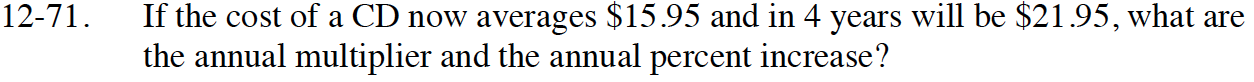Home > CCA2 > Chapter 12 > Lesson 12.1.4 > Problem12-71

12-71.

If the cost of a CD now averages $15.95 and in 4 years will cost$21.95, what are the annual multiplier and the annual percent increase? Homework Help ✎Write an equation of the form y = abx to represent the situation. How does the price change?